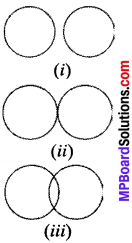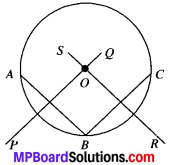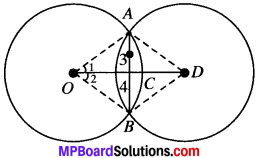# MP Board Class 9th Maths Solutions Chapter 10 Circles Ex 10.3

## MP Board Class 9th Maths Solutions Chapter 10 Circles Ex 10.3

Question 1.
Draw different pairs of circles. How many points does each pair have in common? What is the maximum number of common points?
Solution:
Let us draw different pairs of circles as shown below:We gave,
In figure           Maximum number of common points
(i)             –                     nil
(ii)            –                    one
(iii)           –                    two
Thus, two circles can have at the most two points in common.

Question 2.
Suppose you are given a circle. Give a construction to find its center.
Solution:
Steps of construction:

1. Mark any three points A, B and C on the circle.2. Join AB and BC.
3. Draw the perpendicular bisector of AS. Let it be PQ.
4. Draw the perpendicular bisector of BC. Let it be RS.
5. PQ and RS intersect at point O.
6. O is the center of given circle.Question 3.
If two circles intersect at two points, prove that their centers lie on the perpendicular bisector of the common chord.
Solution:
Given:
Circles C(O, r) and C(D, r1) intersect at A and B.
To prove:
OD is perpendicular bisector of AS.Construction:
Join OA, AD, OB and BD.
Proof:
In ∆AOD and ∆BOD,
OA = OB
DA = DB (Radii of the circle)
OD = OD (Common)
∆AOD ≅ ∆BOD (By SXS)
and ∠1 = ∠2 (By CPCT)
In ∆OAC and ∆OBC,
OA = OB (Radii of the circle)
OC =OC (Common)
∠1 = ∠2 (Proved)
∆AOC = ∆OBC (By SAS)
So ∠3 = ∠4 (By CPCT)
and AC = BC (By CPCT)
∠3 + ∠4 = 180.°
2∠3 = 180°
∠3 = $$\frac{180^{\circ}}{2}$$ =90° (UA’s)
OD is perpendicular bisector of AB.